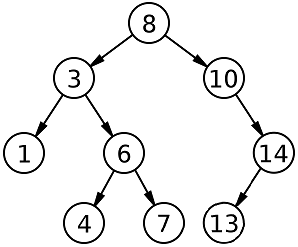New update is available. Click here to update.

# Ceil from BST

Last Updated: 3 Dec, 2020
Difficulty: Easy

## PROBLEM STATEMENT

#### Ninja is given a binary search tree and an integer. Now he is given a particular key in the tree and returns its ceil value. Can you help Ninja solve the problem?

##### Note:
``````Ceil of an integer is the closest integer greater than or equal to a given number.
For example:
arr[] = {1, 2, 5, 7, 8, 9}, key = 3.
The closest integer greater than 3 in the given array is 5. So, its ceil value in the given array is 5.
``````
##### Input Format:
``````The first line of input contains a single integer T, representing the number of test cases.

The first line of each test case contains elements in the level order form. The line consists of values of nodes separated by a single space. In case a node is null, we take -1 in its place.

The second line of each test case contains integer X, denoting the key value.
``````
##### Output Format :
`````` The first and only line of each test case in the output contains ceil of integer X from given BST.
``````
##### Note:
``````You are not required to print the expected output; it has already been taken care of. Just implement the function.
``````
##### Example``````for the above tree
X=2
ceil =3
X=7
ceil =8
X=12
ceil =13
``````
##### Constraints:
``````1 <= T <= 10
1 <= N <= 10^5
0 <= node data <= 10^9
1 <= X <= 10^9

Time limit: 1 second
``````